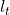## You have two rods, one is made of aluminum and the other is made of Pyrex glass. At T = 0℃, the length of the aluminum rod is LA???? = 3.070

Question

You have two rods, one is made of aluminum and the other is made of Pyrex glass. At T = 0℃, the length of the aluminum rod is LA???? = 3.070 m, and the length of the Pyrex rod is LPyrex = 3.100 m. You are given that the coefficient of linear expansion for aluminum is ????A???? = 24 × 10−6 1 ℃ and ????Pyrex = 3.3 × 10−6 1 ℃ . Please keep 4 significant figures in all your results. a. At what temperature will the aluminum rod and the Pyrex rod have the same length?

in progress 0
2 months 2021-07-15T07:43:33+00:00 1 Answers 0 views 0

Explanation:

The length of rod after rise in temperature can be calculated by the formula= l₀ ( 1 + αΔt)is increased length , l₀ is original length , α  is coefficient of thermal expansion and Δt is rise in temperature.

Let after rise in temperature of Δt in both , they become equal in length

3.07 ( 1 + 24 x 10⁻⁶Δt ) = 3.1 ( 1 + 3.3 x 10⁻⁶ Δt )

3.07 + 73.68 x 10⁻⁶Δt  = 3.1  + 10.23 x 10⁻⁶ Δt

.03 = 63.45 x 10⁻⁶Δt

Δt = 472.8

At 472.8 °C they will have same length .